EarthIntroduction to Atmospheric Science by Prof. C. Balaji (NPTEL):- Lecture 01: Introduction

• 0
• 0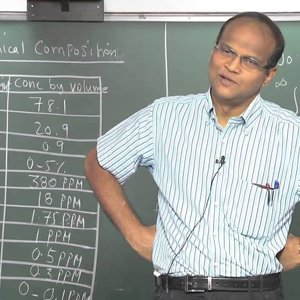Introduction to Atmospheric Science by Prof.C. Balaji (NPTEL):- Lecture 02: Atmosphere - A brief survey (Pressure, Temperature & Chemical composition)

• 0
• 0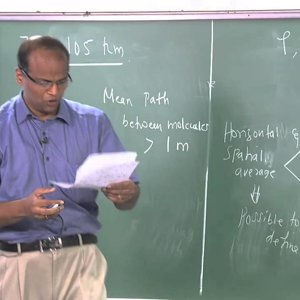Introduction to Atmospheric Science by Prof. C. Balaji (NPTEL):- Lecture 03: Atmosphere-A brief survey (Vertical structure of the atmosphere)

• 0
• 0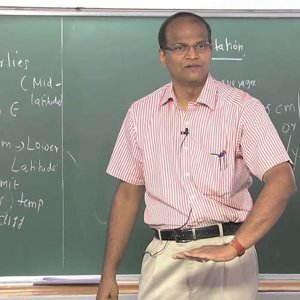Introduction to Atmospheric Science by Prof. C. Balaji (NPTEL):- Lecture 04: Vertical structure of atmosphere and The Earth system - Oceans 1

• 0
• 0Introduction to Atmospheric Science by Prof. C. Balaji (NPTEL):- Lecture 05: The Earth system – Oceans 2 & Marine biosphere

• 0
• 0Introduction to Atmospheric Science by Prof. C. Balaji (NPTEL):- Lecture 06: The Earth system – Hydrological cycle 1

• 0
• 0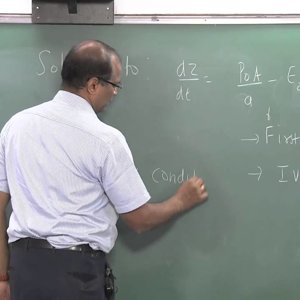Introduction to Atmospheric Science by Prof. C. Balaji (NPTEL):- Lecture 07: The Earth system – Hydrological cycle 2 & Carbon cycle 1

• 0
• 0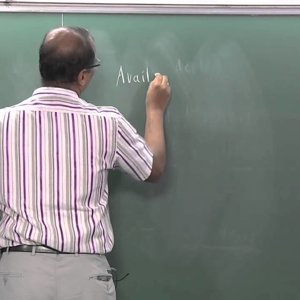Introduction to Atmospheric Science by Prof. C. Balaji (NPTEL):- Lecture 08: The Earth system – Carbon cycle 2 & Carbon in the oceans Earth's crust 1

• 0
• 0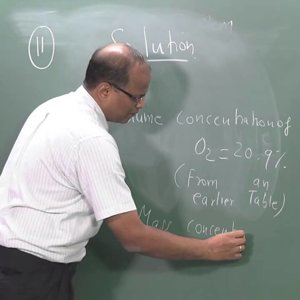Introduction to Atmospheric Science by Prof. C. Balaji (NPTEL):- Lecture 09: The Earth system -- Carbon in the oceans Earth's crust 2

• 0
• 0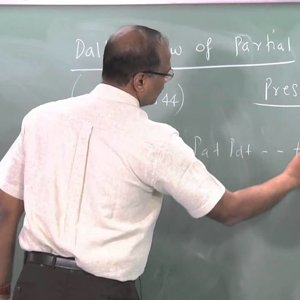Introduction to Atmospheric Science by Prof. C. Balaji (NPTEL):- Lecture 10: Atmospheric Thermodynamics - Introduction

• 0
• 0Introduction to Atmospheric Science by Prof. C. Balaji (NPTEL):- Lecture 11: The hydrostatic equation

• 0
• 0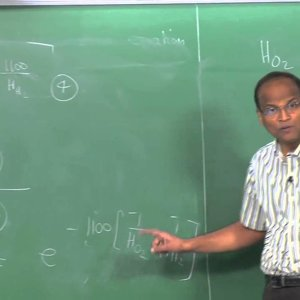Introduction to Atmospheric Science by Prof. C. Balaji (NPTEL):- Lecture 12: Hypsometric equation and pressure at sea level

• 0
• 0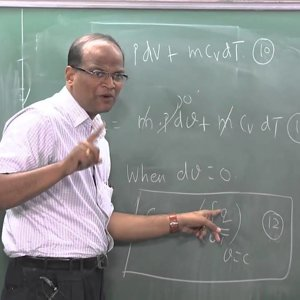Introduction to Atmospheric Science by Prof. C. Balaji (NPTEL):- Lecture 13: Basic Thermodynamics

• 0
• 0Introduction to Atmospheric Science by Prof. C. Balaji (NPTEL):- Lecture 14: Concept of air parcel and dry adiabatic lapse rate

• 0
• 0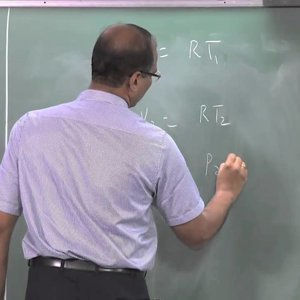Introduction to Atmospheric Science by Prof. C. Balaji (NPTEL):- Lecture 15: Potential temperature

• 0
• 0Introduction to Atmospheric Science by Prof. C. Balaji (NPTEL):- Lecture 16: Skew-T ln-P chart

• 0
• 0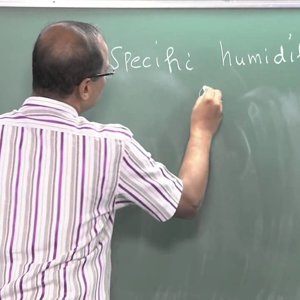Introduction to Atmospheric Science by Prof. C. Balaji (NPTEL):- Lecture 17: Problems using Skew-T ln-P chart 1

• 0
• 0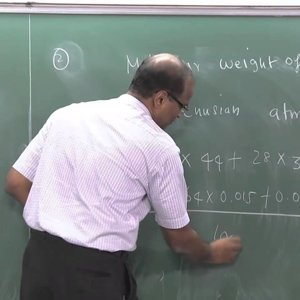Introduction to Atmospheric Science by Prof. C. Balaji (NPTEL):- Lecture 18: Problems using Skew-T ln-P chart 2

• 0
• 0Introduction to Atmospheric Science by Prof. C. Balaji (NPTEL):- Lecture 19: Problems using Skew-T ln-P chart 3

• 0
• 0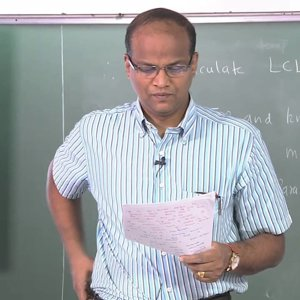Introduction to Atmospheric Science by Prof. C. Balaji (NPTEL):- Lecture 20: Lifting Condensation Level (LCL) 1

• 0
• 0Introduction to Atmospheric Science by Prof. C. Balaji (NPTEL):- Lecture 22: Saturated Adiabatic and Psuedo-adiabatic processes

• 0
• 0Introduction to Atmospheric Science by Prof. C. Balaji (NPTEL):- Lecture 23: Equivalent potential temperature and wet bulb potential temperature

• 0
• 0Introduction to Atmospheric Science by Prof. C. Balaji (NPTEL):- Lecture 24: Normand's rule - Chinook winds

• 0
• 0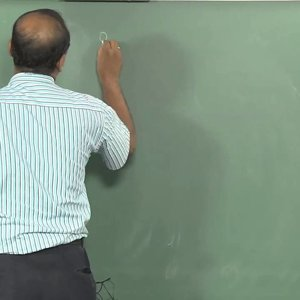Introduction to Atmospheric Science by Prof. C. Balaji (NPTEL):- Lecture 25: Problems on Chinook wind and static stability

• 0
• 0Introduction to Atmospheric Science by Prof. C. Balaji (NPTEL):- Lecture 26: Static stability-Brunt-Visala frequency

• 0
• 0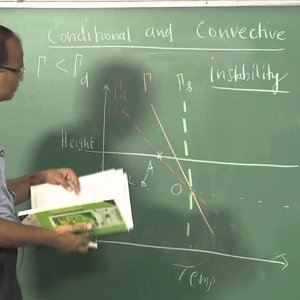Introduction to Atmospheric Science by Prof. C. Balaji (NPTEL):- Lecture 27: Conditional and convective instability

• 0
• 0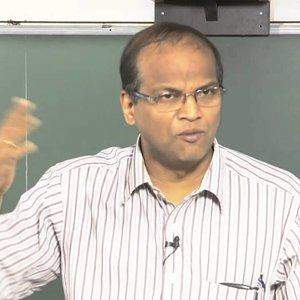Introduction to Atmospheric Science by Prof. C. Balaji (NPTEL):- Lecture 28: Static stability - Problems using radiosonde data and skew T ln P chart

• 0
• 0Introduction to Atmospheric Science by Prof. C. Balaji (NPTEL):- Lecture 29: The second law of thermodynamics – Clausius Clapeyron relation 1

• 0
• 0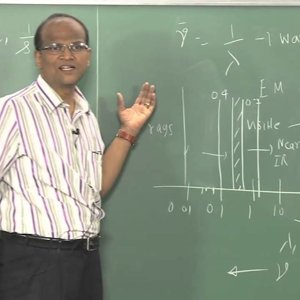Introduction to Atmospheric Science by Prof. C. Balaji (NPTEL):- Lecture 30: Clausius Clapeyron relation 2

• 0
• 0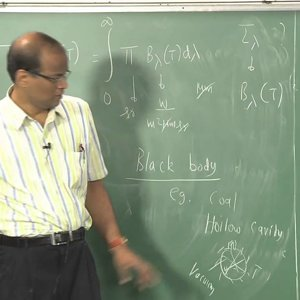Introduction to Atmospheric Science by Prof. C. Balaji (NPTEL):- Lecture 31: Atmospheric radiation – Radiation laws

• 0
• 0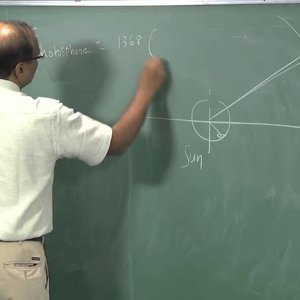Introduction to Atmospheric Science by Prof. C. Balaji (NPTEL):- Lecture 32: Planck's distribution and Inverse square law

• 0
• 0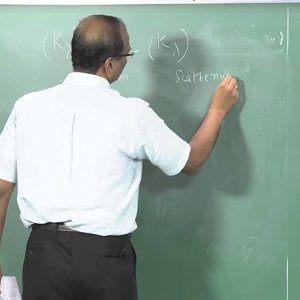Introduction to Atmospheric Science by Prof. C. Balaji (NPTEL):- Lecture 33: Physics of scattering, emmision and absorption 1

• 0
• 0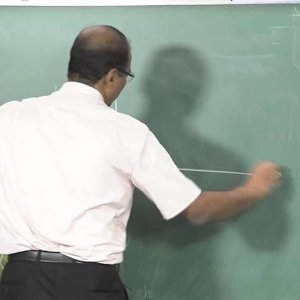Introduction to Atmospheric Science by Prof. C. Balaji (NPTEL):- Lecture 34: Physics of scattering, emmision and absorption 2

• 0
• 0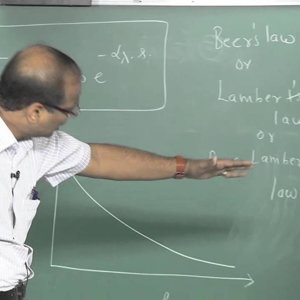Introduction to Atmospheric Science by Prof. C. Balaji (NPTEL):- Lecture 35: Radiative Transfer Equation 1

• 0
• 0Introduction to Atmospheric Science by Prof. C. Balaji (NPTEL):- Lecture 36: Radiative Transfer Equation 2

• 0
• 0Introduction to Atmospheric Science by Prof. C. Balaji (NPTEL):- Lecture 37: Radiative heating profiles of the atmosphere

• 0
• 0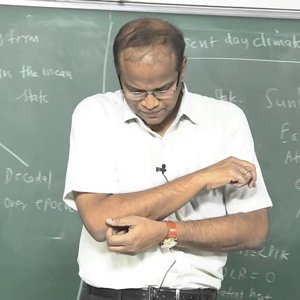Introduction to Atmospheric Science by Prof. C. Balaji (NPTEL):- Lecture 38: Climate Dynamics – Introduction

• 0
• 0Introduction to Atmospheric Science by Prof. C. Balaji (NPTEL):- Lecture 39: Climate sensitivity and feedback

• 0
• 0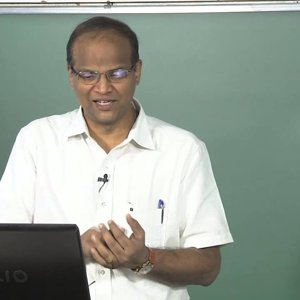Introduction to Atmospheric Science by Prof. C. Balaji (NPTEL):- Lecture 40: Climate change

• 0
• 0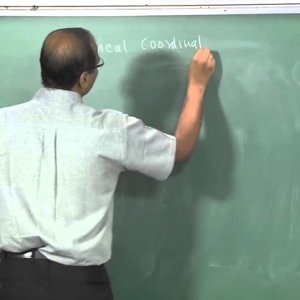Introduction to Atmospheric Science by Prof. C. Balaji (NPTEL):- Lecture 41: Atmospheric dynamics

• 0
• 0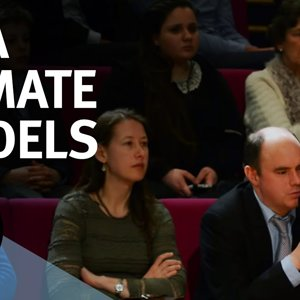• 0
• 0